ABACUS: MYSTERY OF THE BEAD
The Bead Unbaffled - An Abacus Manual

###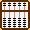Traditional Multiplication techniques for Chinese Suan Pan - The "Extra Bead" and the "Suspended Bead"

The Suan Pan, or Chinese abacus, had been in use in China for two or three centuries before making its journey to Japan sometime in the late 1500's. Unlike the present day Japanese Soroban which has evolved to become an instrument with a 1/4 bead construction, the Suan Pan has retained its classic 2/5 bead construction - 2 beads above the reckoning bar, 5 beads below. The techniques described below may offer some idea as to why this might be so.

These techniques are older and differ from modern Chinese methods in that the order of multiplication and adding products to the frame is different. These techniques might even be recognizable to an older generation in Japan, many of whom grew up learning older methods. New ideas and changes to the physical makeup of the Japanese abacus early in the last century brought about changes in the way many modern day Japanese do their abacus work. In learning these Chinese techniques, not only does it enable a better understanding of abacus use, it also provides a more in-depth look at the instrument and the thought behind its development and use.

• The Suspended Bead technique also uses the top most heaven bead but in this case it's placed ½ way down the rod so that the bead neither touches the frame above nor the bead below; it has a value of 10. (see Example 4: Steps 3 & 4 below)

Before beginning these techniques, it would be useful to have a good understanding of both the addition and multiplication techniques described in: Abacus: Mystery of the Bead

#### The Unit rod and the Decimal

This technique makes working with decimals and the unit rod wonderfully easy. One has only to count either the whole numbers or the trailing zeros in the multiplier then shift the unit rod accordingly.

##### 1.03...... one whole number - unit rod shifts 1 rod to the right. 45.003... two whole numbers - unit rod shifts 2 rods to the right. 0.75....... no whole numbers, no trailing zeros, unit rod does *not* shift. 0.0125... one trailing zero - unit rod shifts 1 rod to the left. 0.003..... two trailing zeros - unit rod shifts 2 rods to the left...etc.
 Suan Pan and the Decimal - Multiplication: Shifting

Traditional Chinese multiplication techniques differ from the Japanese multiplication techniques. Particularly different is the order in which we multiply numbers. Before moving on to the the Extra Bead and the Suspended Bead techniques we'll do two simple examples that will help to illustrate how this traditional multiplication method works.

Example 1: 456 x 23 = 10,488

##### ▪ Rod H x B x A. Note: the multiplicand on rod H changes to become part of the product. ▪ Rod G x B x A. Note: the multiplicand on rod G changes to become part of the product. ▪ Rod F x B x A. Note: the multiplicand on rod F changes to become part of the product.

Step 1: Choose rod H to be the unit rod. Set multiplicand 456 on rods FGH and multiplier 23 on AB. The multiplier has two whole numbers therefore the unit rod will shift two to the right bringing us to rod J. (Fig.1)Fig.1 ```Step 1 A B C D E F G H I J K L M          2 3 0 0 0 4 5 6 0 0 0 0 0 ```

Step 2:
Multiply 6 by 3 and add 18 to rods IJ. (Fig. 2)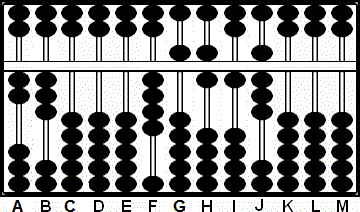Fig.2 ```Step 2 A B C D E F G H I J K L M          2 3 0 0 0 4 5 6 0 0 0 0 0 + 1 8       2 3 0 0 0 4 5 6 1 8 0 0 0 ```

Step 3:
Multiply 6 by 2 and add 12 on rods HI. Note that what was multiplicand 6 on H has now changed to 1. (Fig. 3)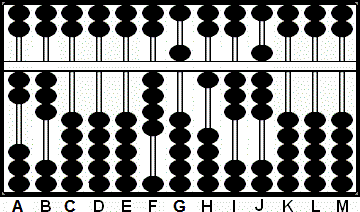Fig.3 ```Step 3 A B C D E F G H I J K L M          2 3 0 0 0 4 5 6 1 8 0 0 0 + (1)2        2 3 0 0 0 4 5 1 3 8 0 0 0 ```

Step 4:
Multiply 5 by 3 and add 15 on to rods HI. (Fig. 4)Fig.4 ```Step 4 A B C D E F G H I J K L M          2 3 0 0 0 4 5 1 3 8 0 0 0 + 1 5        2 3 0 0 0 4 5 2 8 8 0 0 0 ```

Step 5: Multiply 5 by 2 and add 10 to rods GH. Note that what was multiplicand 5 on G has now changed to 1. (Fig. 5)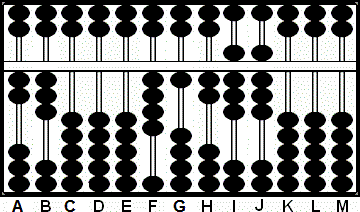Fig.5 ```Step 5 A B C D E F G H I J K L M          2 3 0 0 0 4 5 2 8 8 0 0 0 + (1)0        2 3 0 0 0 4 1 2 8 8 0 0 0 ```

Step 6: Multiply 4 by 3 and add 12 to rods GH. (Fig. 6)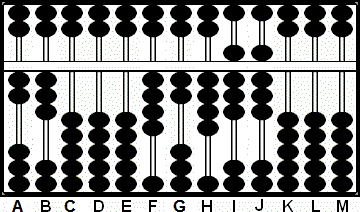Fig.6 ```Step 6 A B C D E F G H I J K L M          2 3 0 0 0 4 1 2 8 8 0 0 0 + 1 2        2 3 0 0 0 4 2 4 8 8 0 0 0 ```

Step 7 and the answer: Multiply 4 by 2 and add 08 to rods FG. Once again note the change; what was originally multiplicand 4 on F changes to 0 and then to 1 after adding 8 to 2 on rod G.  This leaves the answer 10,488 on rods F - J. (Fig. 7)Fig.7 ```Step 7 A B C D E F G H I J K L M          2 3 0 0 0 4 2 4 8 8 0 0 0 + (0)8        2 3 0 0 0 1 0 4 8 8 0 0 0 ```

Example 2: 347 x 276 = 95,772

##### ▪ Rod H x B x C x A. Note: the multiplicand on rod H changes to become part of the product. ▪ Rod G x B x C x A. Note: the multiplicand on rod G changes to become part of the product. ▪ Rod F x B x C x A. Note: the multiplicand on rod F changes to become part of the product.

Step 1: Choose rod H to be the unit rod. Set multiplicand 347 on rods FGH and multiplier 276 on rods ABC. The multiplier has three whole digits therefore the unit rod will shift three rods to the right bringing us to rod K.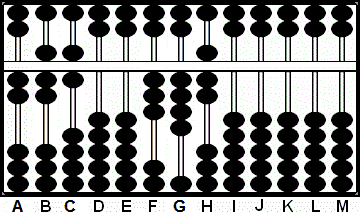Fig.1 ```Step 1 A B C D E F G H I J K L M          2 7 6 0 0 3 4 7 0 0 0 0 0 ```

Step 2: Multiply 7 by 7 and add 49 to rods IJ.
2a: Multiply 7 by 6 and add 42 to rods JK.
2b: Multiply 7 by 2 and add 14 to rods HI. Note that what was multiplicand 7 on H has now changed to 1. (Fig. 2)Fig.2 ```Step 2 A B C D E F G H I J K L M          2 7 6 0 0 3 4 7 0 0 0 0 0 + 4 9 + 4 2 + (1)4         2 7 6 0 0 3 4 1 9 3 2 0 0``` ` `

Step 3: Multiply 4 by 7 and add 28 to rods HI.
3a:
Multiply 4 by 6 and add 24 to rods IJ.
3b:
Multiply 4 by 2 and add 08 to rods GH. Note that what was originally multiplicand 4 on G changes to 0 and then to 1 after adding 8 to rod H. (Fig. 2)Fig.3 ```Step 3 A B C D E F G H I J K L M          2 7 6 0 0 3 4 1 9 3 2 0 0 + 2 8 + 2 4 + (0)8          2 7 6 0 0 3 1 2 9 7 2 0 0 ```

Step 4: Multiply 3 by 7 and add 21 to rods GH.
4a:
Multiply 3 by 6 and add 18 to rods HI.
4b and the answer: Multiply 3 by 2 and add 06 to rods FG. Once again note the change; what was originally multiplicand 3 on F changes to 0. This leaves the answer 95,772 on rods G - K. (Fig. 4)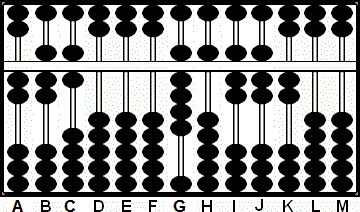Fig.4 ```Step 4 A B C D E F G H I J K L M          2 7 6 0 0 3 1 2 9 7 2 0 0 + 2 1 + 1 8 + (0)6          2 7 6 0 0 0 9 5 7 7 2 0 0 ```

Example 3: "The Extra Bead"

When it comes to adding products onto the abacus, most techniques require the use of complementary numbers. In the examples below we'll use this approach wherever we can. However in Step 3 (see below) using the complementary number might be confusing.

189 x 576 = 108,864

Step 1: Set the multiplier 576 on DEF and the multiplicand 189 on HIJ. (Fig.1)Fig. 1 ```Step 1 A B C D E F G H I J K L M       0 0 0 5 7 6 0 1 8 9 0 0 0 ```

Step 2: Multiply the 9 on rod J by the 7 on E and add the product 63 on KL.

2a: Multiply the 9 on rod J by the 6 on F and add the product 54 on LM

2b: Multiply the same 9 on rod J by the 5 on rod D, remove the 9 on J and place the product 45 on JK . This leaves 185184 on rods H - M. (Fig.2)Fig. 2 ```Step 2 A B C D E F G H I J K L M       0 0 0 5 7 6 0 1 8 9 0 0 0 + 6 3 Step 2 + 5 4 Step 2a (4)5 Step 2b 0 0 0 5 7 6 0 1 8 5 1 8 4 ```

This is where we use we use the Extra Bead. At this point we're going to multiply the 8 on rod I by the 7 on E. If we were to do a carry here then we'd have have to change the 8 to 9. That would be confusing. (Especially since it's the 8 that's going to be multiplied by each of the digits in the multiplier.) Since there is an extra 5 bead available on J we'll use it to avoid confusion.

Step 3:
Multiply the 8 on rod I by the 7 on E and add the product 56 on JK, leaving 1 8 "10" 7 8 4 on rods H - M. (Fig.3)Fig. 3 ```Step 3 A B C D E F G H I J K L M       0 0 0 5 7 6 0 1 8 5 1 8 4 + 5 6 Step 3 0 0 0 5 7 6 0 1 8"10"7 8 4 ```

 * It may be interesting to note that many Japanese Soroban made between circa 1850 and 1930 have one heaven bead and five earth beads. (i.e. 1 bead above the reckoning bar and 5 beads below.)  I've always been confused as to why this might be. Learning from some of these older techniques may provide a clue. In step 3 one could, as an alternative, show the number 10 on rod J as one heaven bead down and 5 earth beads up.*

3a: From here we can continue. Multiply the 8 on rod I by the 6 on F and add the product 48 on KL

3b: Multiply the 8 on rod I by the 5 on D, remove the 8 on I and place the product 40 on rods IJ. This leaves 1 4 "11" 2 6 4 on rods H - M.

3c: Next clear the 10 from rod J and carry it over to rod I changing the 4 to 5. This leaves 151264 on rods H - M. (Fig.4)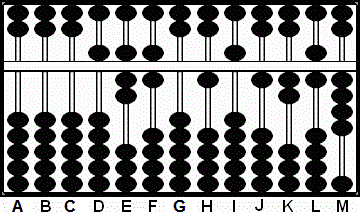Fig. 4 ```Steps 3a-3b-3c A B C D E F G H I J K L M       0 0 0 5 7 6 0 1 8"10"7 8 4 + 4 8 Step 3a + (4)0 Step 3b 0 0 0 5 7 6 0 1 4"11"2 6 4 + extra bead 1 0 Step 3c 0 0 0 5 7 6 0 1 5 1 2 6 4 ```

Step 4: Now we can continue using complements in the normal way. Multiply the 1 on rod H by the 7 on rod E and add the product 7 on rod J

4a: Multiply the 1 on rod H by the 6 on rod F and add the product 6 on rod K

4b & the answer: Multiply the same 1 on rod H by the 5 on rod D, remove the 1 on rod H and add the product 5. This leaves 108,864 on rods H - M, which is the answer. (Fig.5)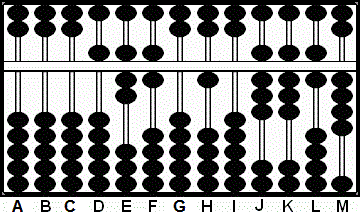Fig. 5 ```Step 4 A B C D E F G H I J K L M       0 0 0 5 7 6 0 1 5 1 2 6 4 + 0 7 Step 4 + 0 6 Step 4a + 0 5 Step 4b 0 0 0 5 7 6 0 1 0 8 8 6 4```

Example 4: "The Suspended Bead" (Xuan - zhu)

In this next example we'll use a technique where we bring down the upper most 5 bead ½ way down the rod so that it touches neither the frame above nor the bead below. This Suspended Bead has a value of 10. Once again in solving this next example, we'll use complementary numbers as much as possible. However, in two instances it will be clear that having extra beads simplifies the process.

It should be noted that while it's fun to do a problem like this it's a fairly extreme example and instances where one needs the suspended bead are quite rare.

989 x 898 = 888, 122

Step 1: Set the multiplier 898 on DEF and the multiplicand 989 on HIJ. (Fig.6)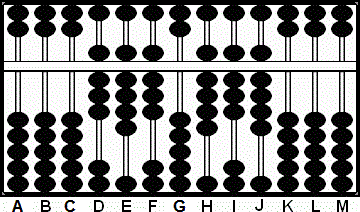Fig. 6 ```Step 1 A B C D E F G H I J K L M       0 0 0 8 9 8 0 9 8 9 0 0 0 ```

Step 2: Multiply the 9 on rod J by the 9 on E and add the product 81 on KL.

2a: Multiply the 9 on rod J by the 8 on F and add the product 72 on LM

2b: Multiply the same 9 on rod J by the 8 on rod D, remove the 9 on J and place product 72 on JK . This leaves 988082 on rods H - M. Fig.7)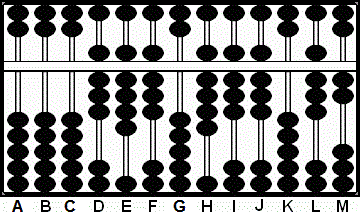Fig. 7 ```Step 2 A B C D E F G H I J K L M       0 0 0 8 9 8 0 9 8 9 0 0 0 + 8 1 Step 2 + 7 2 Step 2a (7)2 Step 2b 0 0 0 8 9 8 0 9 8 8 0 8 2 ```

This is where we use we use the Suspended Bead. At this point we're going to multiply the 8 on rod I by the 9 on E. If we were to do a carry here then we'd have have to change the 8 to 9. That would be confusing. (Especially since it's the 8 that's going to be multiplied by each of the digits in the multiplier.) We might loose track. Instead we use the "Suspended Bead" technique.

Step 3: Multiply the 8 on rod I by the 9 on E and add the product 72 on JK. In bringing down the suspended bead to signify 10 and subtracting 3 we've added 7 to rod J giving the rod a total value of 15.

3a: Multiply the 8 on rod I by the 8 on F and add the product 64 on KL. This leaves a value of 9 8 "15" 9 2 2 on rods H - M. (Fig.8)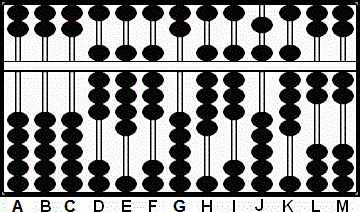Fig. 8 ```Step 3-3a A B C D E F G H I J K L M       0 0 0 8 9 8 0 9 8 8 0 8 2 + 7 2 Step 3 + 6 4 Step 3a 0 0 0 8 9 8 0 9 8"15"9 2 2 ```

3b: Multiply the same 8 on rod I by the 8 on rod D, remove the 8 on I and place the product 64 on IJ . This leaves 9 6 "19" 9 2 2 on rods H - M.

3c: Next clear the "Suspended" 10 from rod J and carry it over to rod I changing the 6 to 7. This leaves 979922 on rods H - M. (Fig.9)Fig. 9 ```Step 3b-3c A B C D E F G H I J K L M       0 0 0 8 9 8 0 9 8"15"9 2 2 (6)4 Step 3b 0 0 0 8 9 8 0 9 6"19"9 2 2 + suspended 1 0 Step 3c 0 0 0 8 9 8 0 9 7 9 9 2 2 ```

Step 4: Multiply the 9 on H by the 9 on E and add the product 81 on IJ using the Suspended Bead technique.

4a: Multiply the 9 on H by the 8 on F and add the product 72 on JK. This leaves 9 "16" 8 1 2 2 on HIJKLM. (Fig.10)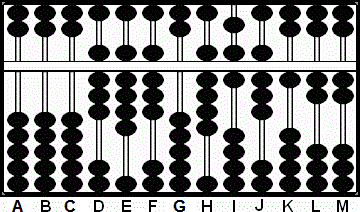Fig. 10 ```Steps 4-4a A B C D E F G H I J K L M       0 0 0 8 9 8 0 9 7 9 9 2 2 + 8 1 Step 4 + 7 2 Step 4a 0 0 0 8 9 8 0 9"16"8 1 2 2 ```

4b: Multiply the 9 on H by the 8 on D, remove the 9 on H and place the product 72 on HI. This leaves 7 "18" 8 1 2 2 on rods H - M.

4c & the answer: Next clear the "Suspended" 10 from rod I and carry it over to rod H changing the 7 to 8. This leaves 888122 on rods H - M, which is the answer. (Fig.11)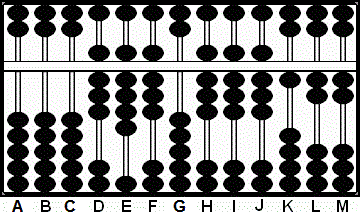Fig. 11 ```Step 4b-4c A B C D E F G H I J K L M       0 0 0 8 9 8 0 9"16"8 1 2 2 (7)2 Step 4b 0 0 0 8 9 8 0 7"18"1 2 2 + suspended 1 0 Step 4c 0 0 0 8 9 8 0 8 8 8 1 2 2 ```

Over the centuries both the Japanese and the Chinese have developed and used many different techniques for solving problems of multiplication on the abacus. One widely used method is the one illustrated in the 1954 book, "The Japanese Abacus, its use and Theory" by Takashi Kojima. When introducing his technique, even Kojima says, "There are several methods of multiplication on the abacus. The one introduced in the following pages is a recent method..."

Even though it's possible to solve multiplication problems while using these Chinese techniques on a 1/4 bead Soroban, the above examples show the answers come more easily with the help of extra beads.

June, 2004
Totton Heffelfinger  Toronto Ontario  Canada

Email
totton[at]idirect[dot]com